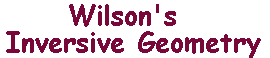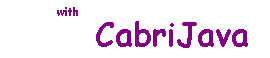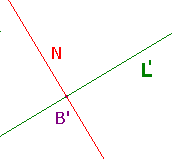Orthogonal i-lines

 The Uniqueness Theorem If A and B are distinct points on an i-line L, then there is a unique i-line M through A and B orthogonal to C. Since the proof is a good example of the use of inversion, we give it in full. Proof Since A and B are distinct, one of them (A say) is not Ñ. Let C be a circle with centre A, so L passes through the centre of C. Let M be any i-line through A and B. Let B', L', M' be the inverses of B, L, N with respect to C. By the Invariance Theorem, L and M are orthogonal if and only if L' and M' are. By the Inversion Theorem, L' and M' are extended lines. As B is on L and M, the inverses pass through B'. But there is exactly one i-line, N say, through B' perpendicular to L'. Hence there is a unique M (the inverse of N with respect to C), as required. Note the structure of the proof. We transform the picture to get a single, easy case. We verify that the result in the special case implies the result in the original picture.In a similar way we can prove a result fundamental to the creation of hyperbolic geometry. The Mirror Theorem Suppose that L and M are i-lines, then iL(M) = M if and only if L and M are orthogonal.